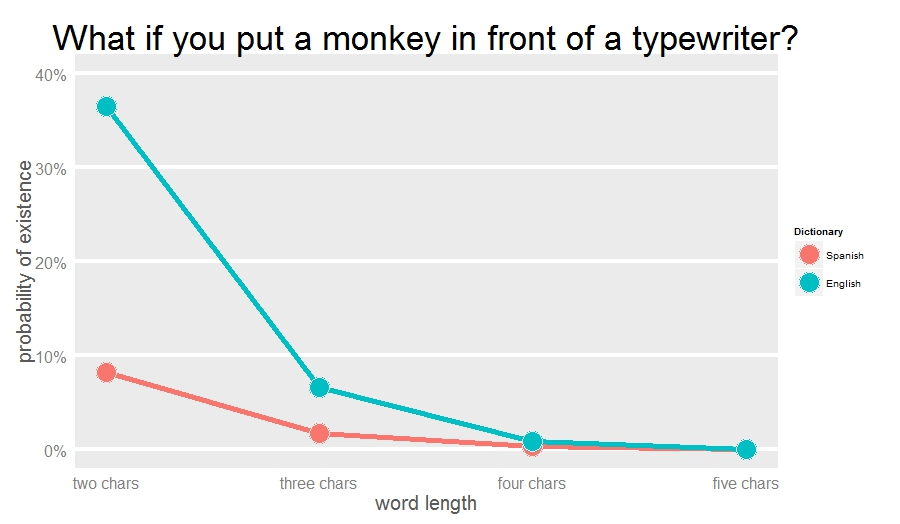# Shakespeare Is More Monkey-Friendly Than Cervantes

Ford, there is an infinite number of monkeys outside who want to talk to us about this script for Hamlet they have worked out (from Episode 2 of The Hitchhiker’s Guide to the Galaxy by Douglas Adams)

Some days ago I was talking with a friend about the infinite monkey theorem which is a funny interpretation of what thinking-in-infinite can produce. The same day, in my weekly English class, my teacher said that Anglo-saxon words do tend to be short, very often monosyllabic such as function words such as to, of, from etc and everyday words such as go, see run, eat, etc.

Both things made me think that a monkey could have easier to type a Shakespeare text rather than a Cervantes one. I cannot imagine a definitive way to demonstrate this but this experiment support my hypothesis. After simulating random words of 2, 3, 4 and 5 characters I look for them in English(1) and Spanish(2) dictionaries, which I previously downloaded from here. Result: I find more random words in the English one. These are the results of my experiment:For example, around 38% of two-chars words match with English dictionary and only 9% with Spanish one. This is why I think that, in the infinite, I would be easier for a monkey to replicate a Shakespeare text than a Cervantes one.

Here you have the code:

```library(ggplot2)
library(scales)
df.lang=do.call("rbind", list(esp.dic, eng.dic))
df.lang\$WORD=tolower(iconv(df.lang\$WORD, to="ASCII//TRANSLIT"))
df.lang=unique(df.lang)
results=data.frame(LANG=character(0), OCCURRENCES=numeric(0), SIZE=numeric(0), LENGTH=numeric(0))
for (i in 2:5)
{
df.monkey=data.frame(WORD=replicate(20000, paste(sample(c(letters), i, replace = TRUE), collapse='')))
results=rbind(results, data.frame(setNames(aggregate(WORD ~ ., data = merge(df.lang, df.monkey, by="WORD"), FUN=length), c("LANG","OCCURRENCES")), SIZE=20000, LENGTH=i))
}
opt=theme(panel.background = element_rect(fill="gray92"),
panel.grid.minor = element_blank(),
panel.grid.major.x = element_blank(),
panel.grid.major.y = element_line(color="white", size=1.5),
plot.title = element_text(size = 35),
axis.title = element_text(size = 20, color="gray35"),
axis.text = element_text(size=16),
axis.ticks = element_blank(),
axis.line = element_line(colour = "white"))
ggplot(data=results, aes(x=LENGTH, y=OCCURRENCES/SIZE, colour=LANG))+
geom_line(size = 2)+
scale_colour_discrete(guide = FALSE) +
geom_point(aes(fill=LANG),size=10, colour="gray92",pch=21)+
scale_x_continuous("word length", labels=c("two chars", "three chars", "four chars", "five chars"))+
scale_y_continuous("probability of existence", limits=c(0, 0.4), labels = percent)+
labs(title = "What if you put a monkey in front of a typewriter?")+
opt + scale_fill_discrete(name="Dictionary", breaks=c("ESP", "ENG"), labels=c("Spanish", "English"))
```

(1) The English dictionary was originally compiled from public domain sources
for the amSpell spell-checker by Erik Frambach e-mail: e.h.m.frambach@eco.rug.nl
(2) The Spanish dictionary has been elaborated by Juan L. Varona, Dpto. de Matematicas y Computacion, Universidad de La Rioja, Calle Luis de Ulloa s/n, 26004 SPAIN e-mail: jvarona@siur.unirioja.es

## 4 thoughts on “Shakespeare Is More Monkey-Friendly Than Cervantes”

1.Troy says:

Interesting, but I don’t think so – surely the theorem works on the basis of the monkey typing one letter at a time?

So if you ignore the spaces, surely it depends much more on:
1) Primarily : total number of letters in the work in question, and
2) Secondarily the number of letters in the language/alphabet of the work.

So as an extreme example (excluding spaces):

Macbeth – English – 26 letters – ~ 85 000 characters

Don Quijote – Spanish – 29 letters, – ~ 1 700 000 characters

Macbeth chance: 1 in 26^85 000

Don Quijote chance 1 in 29^1 700 000

to equalize bases 26^85000 = (roughly) 29^82250

Therefore Don Quijote would be ~ 29^1 617 750 TIMES less likely to pop out than Macbeth, no?

1.aschinchon says:

Totally agree, Macbeth sounds much easier. Title is just a way to illustrate the experiment between looking for random words in both dictionaries. Thanks for your comment.

2.TH says:

Is a monkey more likely to hit the space bar than other keys? It’s bigger? Are they more likely to hit the keys in the middle of the keyboard than at the edge? The experiment assumes all keys are equally likely, but I don’t know if that’s a reasonable assumption.

1.aschinchon says:

Since I simulate isolate words I don’t need to take care about space bar. I agree not all keys are equally likely. Mine is an approach which serves as reasonable argument to support my hypothesis. Thanks for your comment!